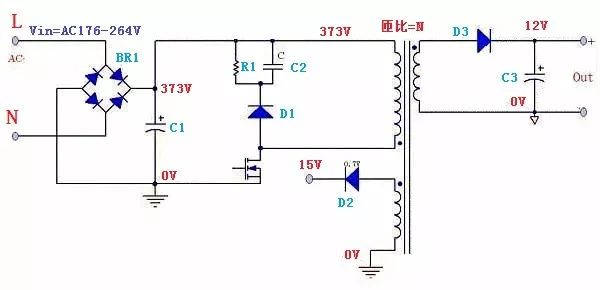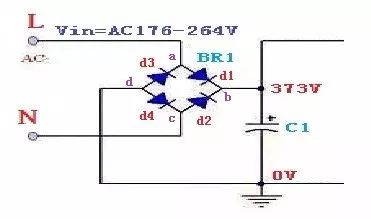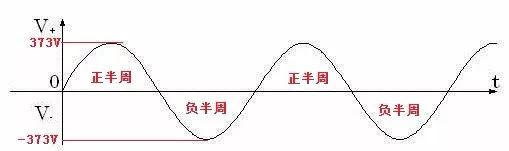﻿ 【ASEMI】Voltage Stress Analysis of "Key Components" of SMPS

### 【ASEMI】Voltage Stress Analysis of "Key Components" of SMPS

Bridge RectifierVoltage Stress Analysis of "Key Components" of SMPSBefore setting up the test and calculation, first set it up, the circuit diagram is designed as above, the main parameters are as follows:

Input voltage: Vin=AC176-264V

Output voltage: Vout=12V

Vcc voltage: Vcc=15V

Transformer turns ratio: N

The stress calculation is performed on the rectifier bridge components in the picture below.The rectifier bridge is composed of four diodes as shown in the figure: d1, d2, d3, d4

Should be bad when the input voltage max, that is, Vin = 264Vac

Therefore, the voltage at both ends of C1 is 373V.

The input voltage waveform is as follows:When the input voltage is in the positive half cycle, the diodes d1 and d4 in the BR1 body are not subjected to high voltage, d2 and d3 are cut off to withstand high voltage, d2 voltage stress is b point potential minus c point potential, d3 voltage stress is a point potential Subtract the d point potential.

The potentials of the positive half-cycles a, b, c, and d are 373V, 373V, 0V, 0V with respect to the large capacitance.

D2 stress = b-c = 373V - 0V = 373V

D3 stress = a-d = 373V-0V = 373V

When the input voltage enters the negative half cycle, the stress of d1 and d4 is 373V.

That is, the working stress of the rectifier bridge in the circuit is 373V.

Since the switching power supply needs to do the lightning surge test, the general rectifier bridge selects the 1000V rectifier bridge.

ASEMI    TECHwill be better

Tag：ASEMI SMPS Prev：ASEMI brand rectifier bridge 3SRB5016 Next：【ASEMI】How to grasp the output waveform of the rectifier bridge?

## News Center

### ASEMI Publication

####Hotline

400-9929-667